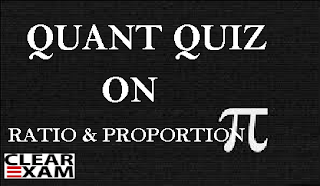## Quant Quiz on Ratio and Proportion for DSSSB Exam

1. If A, B, C and D are four numbers such that A: B = 2 : 3, B: C = 4: 5, C : D = 5: 8 Then, A : D is equal to.
(a)  1 : 3                                (b)  3 : 1
(c)  2 : 3                                (d)  3 : 2

2. If A : B = 3 :4, B : C = 5 : 7 and C : D = 8 : 9. Then the ratio A : D is -
(a)  3 : 7                                (b)  7 : 3
(b)  21 : 10                            (d)  10 : 21

3. If a/b=7/9 and b/c=3/5 Then, the value of a: b: cis
(a)  7 : 9 : 15                         (b)  7 : 9 : 5
(c)  21 : 35 : 45                     (d)  7 : 3 : 15

4. The sum of three numbers is 58. The ratio of first to second is 4: 9 and the second to third is 9: 16, the sum of first and third numbers is  -
(a)  40                                   (b)  29
(c)  28                                   (d)  50

5.  Two numbers are in the ratio 3:  5. If 9 is subtracted from each, the new numbers are in the ratio of 12: 13. Then, smaller number is -
(a)  27                                   (b)  33
(c)  49                                   (d)  55

6.  The mean proportional between the numbers 8 and 18 is
(a)  32/9                                (b)  12
(c)  13                                   (d)  None of these

7.  Find the third proportional to 9 and 12.
(a)  12                                   (b)  14
(c)  16                                   (d)  18

8.  Find the fourth proportional to 4, 5 and 12.
(a)  13                                   (b)  14
(c)  15                                   (d)  16

9.  A bag contains Rs. 102 in the form of rupee, 50 paise and 10 paise coins in the ratio 3: 4: 10. The number of 10 paise coins is -
(a)  340                                 (b)  60
(c)  80                                   (d)  170

10. A sum of Rs. 53 is divided amongst A, B and C in such a way that A gets Rs. 7 more than B gets and B gets Rs. 8 more than C gets, the ratio of their shares is-
(a)  6 : 7 : 8                           (b)  25 : 18 : 10

(c)  12: 14: 9                       (d)  None of these

1. (a) 2. (d) 3. (c) 4. (a) 5. (b) 6. (b) 7. (c) 8. (c) 9. (d) 10. (b)

Thanks

Team Clear CtetWant to Know More
Please fill in the details below:

Name

ltr
item
Clear CTET - Coaching Institute for CTET, DSSSB and KVS: Quant Quiz on Ratio and Proportion for DSSSB Exam
Quant Quiz on Ratio and Proportion for DSSSB Exam
Attempt some Quant Quiz based on Ratio and Proportion for DSSSB Exam
https://1.bp.blogspot.com/-X8G5aw3WUnA/WctM44lbOvI/AAAAAAAAAcc/XyvGYQ2kS80o6g4sQyuVnVOI0vv-8lxsQCLcBGAs/s320/QUANT%2BQUIZ%2BON%2BRATIO%2BFOR%2BCTET.png
https://1.bp.blogspot.com/-X8G5aw3WUnA/WctM44lbOvI/AAAAAAAAAcc/XyvGYQ2kS80o6g4sQyuVnVOI0vv-8lxsQCLcBGAs/s72-c/QUANT%2BQUIZ%2BON%2BRATIO%2BFOR%2BCTET.png
Clear CTET - Coaching Institute for CTET, DSSSB and KVS
https://www.clearctet.com/2017/09/quant-quiz-on-ratio-and-proportion-for.html
https://www.clearctet.com/
https://www.clearctet.com/
https://www.clearctet.com/2017/09/quant-quiz-on-ratio-and-proportion-for.html
true
5140436504468951946
UTF-8